﻿ 开合式电流传感器的补偿方法探讨 Exploration of the Compensation Method of Open-Close Current Sensor

Journal of Sensor Technology and Application
Vol.06 No.02(2018), Article ID:24601,9 pages
10.12677/JSTA.2018.62009

Exploration of the Compensation Method of Open-Close Current Sensor

Shuhong Hu1, Jiale Tian1, Shun Li1, Miaoli Lv1, Di Tang1, Wenjia Shi1, Jinrong Wang1, Junming Tang2

1State Grid Zhejiang Electric Power Company Research Institute, Hangzhou Zhejiang

2Longshine Technology Co., Ltd., Wuxi Jiangsu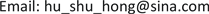Received: Apr. 6th, 2018; accepted: Apr. 20th, 2018; published: Apr. 27th, 2018ABSTRACT

This paper analyzes the error factors of open-close current sensor and presents three compensation methods, and the compensation schematic diagram is given. In addition, this paper introduces an adaptive compensation method based on control theory, which uses a two-stage sensor structure and introduces Input and output feedback. The method implements the adaptive compensation by adjusting the gain ratio automatically after detecting the iron core working point. High precision and good linearity can be achieved in full range.

Keywords:Open-Close, Current Sensor, Compensation Method, Adaptive

1国网浙江省电力公司电力科学研究院，浙江 杭州

2朗新科技股份有限公司，江苏 无锡1. 引言Figure 1. The high precision current sensor with open-type structure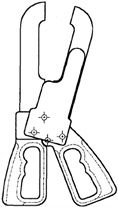Figure 2. The clamp current sensor

2. 开合式电流传感器的误差分析

2.1. 开合式电流传感器的等效电路模型

2.2. 开合式电流传感器的误差分析

${n}_{1}{i}_{1}-{n}_{2}{i}_{2}={n}_{2}{i}_{0}$ (1)

${i}_{0}=\frac{{n}_{1}}{{n}_{2}}{i}_{1}-{i}_{2}$ (2)

${\stackrel{˙}{I}}_{0}={{\stackrel{˙}{I}}^{\prime }}_{1}-{\stackrel{˙}{I}}_{2}$ (3)

$\epsilon =\frac{{\stackrel{˙}{I}}_{0}}{{{\stackrel{˙}{I}}^{\prime }}_{1}}=\frac{{Z}_{2}+{Z}_{L}}{{Z}_{2}+{Z}_{L}+{Z}_{M}}=f+j\delta$ (4)

$\epsilon =\frac{{\stackrel{˙}{I}}_{0}}{{{\stackrel{˙}{I}}^{\prime }}_{1}}=\frac{{Z}_{2}+{Z}_{L}}{{Z}_{2}+{Z}_{L}+{Z}_{M}}$ (5)

${Z}_{M}=jw\frac{u{n}_{2}^{2}s}{l}$ (6)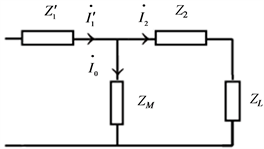Figure 3. The equivalent circuit of open-close current sensor

1) 既然励磁电流是误差的来源，可以直接提取励磁电流，将其叠加到二次负载上，那么二次负载上的电流就没有误差；

2) 励磁电流是电流传感器工作的根本原因，并不能完全消除励磁电流，如果通过外部电路来提供励磁电流，那么等效电路中折算到二次侧的一次电流就会等于二次电流，二次电流将没有误差；

3) 为了降低开合式电流传感器的误差，可以通过降低激磁阻抗两端的电压来实现，那么在二次回路中提供一个适当的电动势，使激磁阻抗两端的电压基本上为零，也可以提高开合式电流传感器的精度。

3. 基于开合式电流传感器原理的补偿方法

3.1. 直接补偿二次电流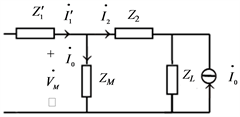Figure 4. The schematic diagram of compensating secondary current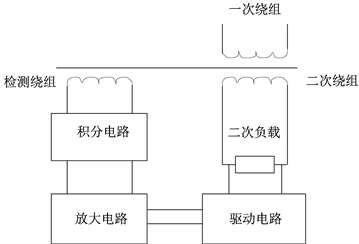Figure 5. The structure of compensating secondary current

${u}_{感应电压}=un\frac{s}{l}\frac{\text{d}{i}_{励磁电流}}{\text{d}t}$ (7)

3.2. 提供励磁电流的补偿方法

3.3. 提供二次电动势的补偿方法

${\stackrel{˙}{I}}_{0}=\frac{{\stackrel{˙}{V}}_{M}}{{Z}_{M}}$ (8)

${\stackrel{˙}{V}}_{M}={\stackrel{˙}{I}}_{2}\left({Z}_{2}+{Z}_{M}\right)$ (9)

${\stackrel{˙}{V}}_{C}\approx {\stackrel{˙}{I}}_{2}\left({Z}_{2}+{Z}_{L}\right)$ (10)

${\stackrel{˙}{V}}_{M}\approx 0$ (11)

${\stackrel{˙}{V}}_{M}={\stackrel{˙}{I}}_{2}\left({Z}_{L}+{Z}_{x}\right)-{\stackrel{˙}{I}}_{3}{Z}_{x}$ (12)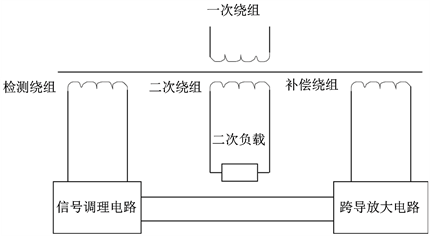Figure 6. The structure of compensating excitation current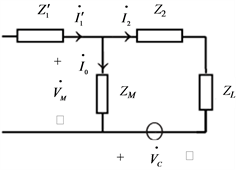Figure 7. The schematic diagram of compensating secondary electromotive force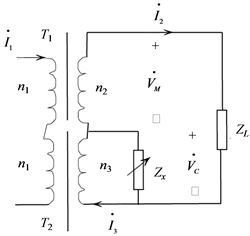Figure 8. The structure of compensating secondary electromotive force

${n}_{2}{\stackrel{˙}{I}}_{2}\approx {n}_{3}{\stackrel{˙}{I}}_{3}$ (13)

${Z}_{x}\approx \frac{{n}_{3}}{{n}_{2}-{n}_{3}}{Z}_{L}$ (14)

4. 引入控制理论的自适应补偿

4.1. 基于输出反馈的参考输入反馈原理

4.2. 在原理性补偿的基础上引入参考输入反馈的补偿方法

1) 首先，运用原理性补偿将开合式电流传感器的误差降到一定范围；

2) 然后，对原理性补偿的开合式电流传感器引入参考输入反馈的补偿方法。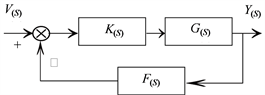Figure 9. The schematic diagram based on Input and output feedback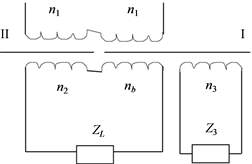Figure 10. The two-stage sensor structure

${n}_{1}{i}_{0}={n}_{1}{i}_{1}-{n}_{2}{i}_{2}$ (15)

${u}_{感应电压}=u{n}_{检测绕组}{n}_{1}\frac{s}{l}\frac{\text{d}{i}_{0}}{\text{d}t}$ (16)

4.3. 引入控制理论的自适应补偿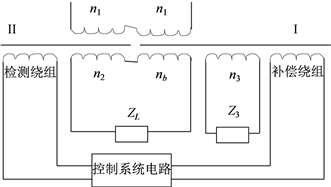Figure 11. The structure based on Input and output feedback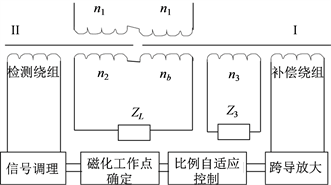Figure 12. The daptive structure

5. 总结

Exploration of the Compensation Method of Open-Close Current Sensor[J]. 传感器技术与应用, 2018, 06(02): 77-85. https://doi.org/10.12677/JSTA.2018.62009

1. 1. Ramboz, J.D. (1996) A Highly Accurate, Hand-Held Clamp-On Current Transformer. IEEE Transactions on Instrumentation and Measurement, 45, 445-448. https://doi.org/10.1109/19.492764

2. 2. 李群, 杨以涵, 李士琨. 电流传感器误差补偿装置的研制[J]. 电网技术, 1996(8): 38-40.

3. 3. 李群, 杨以涵. 电流传感器的外部有源补偿方法[J]. 供用电, 1998(4): 20-21.

4. 4. 李群, 黄伟, 杨以涵, 李蕾. 电流传感器有源补偿方法[J]. 华北电力技术, 1997(2): 25-26.

5. 5. Slomovitz, D. and Santos, A. (2012) A Self-Calibrating Instrument Current Transformer. Measurement, 45, 2213-2217. https://doi.org/10.1016/j.measurement.2012.05.001

6. 6. Betts, P.J. (1983) Two-Stage Current Transformers in Differential Calibration Circuits. IEE Proceedings A Physical Science, Measurement and Instrumentation, Management and Education, Reviews, 130, 324-328. https://doi.org/10.1049/ip-a-1.1983.0058

7. 7. 赵修民. 0.002级双级电流传感器的研制[J]. 仪表技术, 1998(8): 12-14.

8. 8. 赵修民. 升流双级电流传感器[J]. 变压器, 1979(3): 22-25.

9. 9. 双级式0.02级标准电流传感器[J]. 电测与仪表, 1968(Z4): 19-24.

10. 10. 刘君, 吴广宁, 周利军, 等. 零磁通传感器的研究[J]. 电力自动化设备, 2009(8): 67-70.